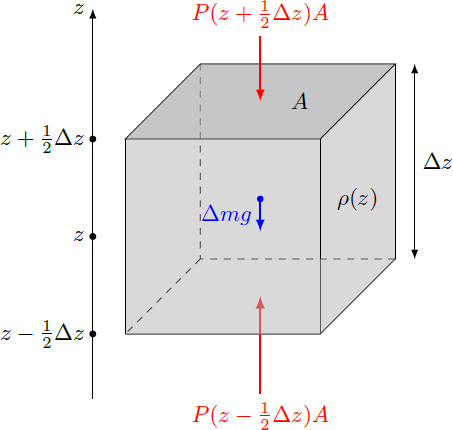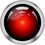# Atmospheric physics 3: Hydrostatic equation

In fact, the atmosphere consists of compressible gases, so that the mass density $\rho(z)$ of the air decreases continuously with the altitude $z$. However, the weight of the gas layers must be supported by the pressure gradient $\frac{dP}{dz}$ in the atmosphere. The balance of compressive forces and gravitation is expressed by the hydrostatic equation: $\frac{dP(z)}{dz} = -\rho(z) g$ with the gravitational acceleration $g \approx 9.81 \,\text{m}/\text{s}^2$.This equation can be derived as follows: We consider a cuboid volume element of the atmosphere with the base area $A$ parallel to the Earth's surface and the height $\Delta z$ (see the diagramm on the right). The mass of this volume is $\Delta m \approx \rho(z) A \Delta z$, so that a weight $\Delta F_g = -\Delta m g$ acts on the entire volume. In addition, the pressures $P(z + \frac{1}{2} \Delta z)$ and $P(z - \frac{1}{2}\Delta z)$ act respectively on the top and bottom of the cuboid. In the static case, the sum of all forces on the volume element must be zero. In the limit $\Delta z \to 0$, the pressure difference changes into the derivative $\frac{dP}{dz}$, so that the difference equation becomes a differential equation. \begin{aligned} \sum F = 0 &= - \Delta m g - P(z + \tfrac{1}{2} \Delta z)A + P(z + \tfrac{1}{2} \Delta z)A \\ &= -\left(\rho(z) g - \frac{P(z + \tfrac{1}{2} \Delta z)- P(z + \tfrac{1}{2} \Delta z)}{\Delta z} \right) A \Delta z \\ &\stackrel{\Delta z \to 0}{\to} -\left(\rho(z) g - \frac{d P(z)}{dz} \right) dV \end{aligned} Since the expression in parentheses must be zero it follows $\boxed{\dfrac{d P(z)}{dz} = - \rho(z) g }$Note by Markus Michelmann
3 years, 5 months ago

This discussion board is a place to discuss our Daily Challenges and the math and science related to those challenges. Explanations are more than just a solution — they should explain the steps and thinking strategies that you used to obtain the solution. Comments should further the discussion of math and science.

When posting on Brilliant:

• Use the emojis to react to an explanation, whether you're congratulating a job well done , or just really confused .
• Ask specific questions about the challenge or the steps in somebody's explanation. Well-posed questions can add a lot to the discussion, but posting "I don't understand!" doesn't help anyone.
• Try to contribute something new to the discussion, whether it is an extension, generalization or other idea related to the challenge.

MarkdownAppears as
*italics* or _italics_ italics
**bold** or __bold__ bold
- bulleted- list
• bulleted
• list
1. numbered2. list
1. numbered
2. list
Note: you must add a full line of space before and after lists for them to show up correctly
paragraph 1paragraph 2

paragraph 1

paragraph 2

[example link](https://brilliant.org)example link
> This is a quote
This is a quote
    # I indented these lines
# 4 spaces, and now they show
# up as a code block.

print "hello world"
# I indented these lines
# 4 spaces, and now they show
# up as a code block.

print "hello world"
MathAppears as
Remember to wrap math in $$ ... $$ or $ ... $ to ensure proper formatting.
2 \times 3 $2 \times 3$
2^{34} $2^{34}$
a_{i-1} $a_{i-1}$
\frac{2}{3} $\frac{2}{3}$
\sqrt{2} $\sqrt{2}$
\sum_{i=1}^3 $\sum_{i=1}^3$
\sin \theta $\sin \theta$
\boxed{123} $\boxed{123}$